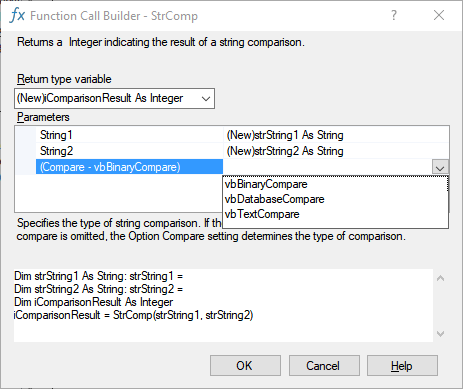﻿ Checking if two strings are equal

# Checking if two strings are equal

Comparing two strings for equality can be tricky. The result of var1 = var2 where Var1 and var2 only differ in the use of capitals depends on the Option Compare Setting. This is risky business indeed. Setting Option Compare is a module level decision effecting statement level results. If a module doesn't include an Option Compare statement, the default text comparison method is Binary (terms defined below):

"abc" = "ABC" » False (Compare Binary)

However, this is not always what one wants. If for example in Excel one wants to check if a workbook with a certain name is open then one wants

"MyWorkbook.xls" = "myworkbook.xls" » True (Compare text)

The way to ensure the required 'compare text'  behavior is by using StrComp function as below.

(StrComp(Str1, Str2, vbTextCompare) = 0)

e.g.
(StrComp("abc", "ABC", vbTextCompare) = 0) » True
(StrComp("abc", "abc", vbTextCompare) = 0)  » True
(StrComp("abc", "ab", vbTextCompare) = 0)   » False(text below copied from VBA Help)

Compare Binary results in string comparisons based on a sort order derived from the internal binary representations of the characters. In Microsoft Windows, sort order is determined by the code page. A typical binary sort order is shown in the following example:

``A < B < E < Z < a < b < e < z < À < Ê < Ø < à < ê < ø``

Compare Text results in string comparisons based on a case-insensitive text sort order determined by your system's locale. When the same characters are sorted using Option Compare Text, the following text sort order is produced:

``(A=a) < (À= à) < (B=b) < (E=e) < (Ê= ê) < (Ø = ø) < (Z=z)``

Option Compare Database can only be used within Microsoft Access. This results in string comparisons based on the sort order determined by the locale ID of the database where the string comparisons occur.

```StrComp(string1, string2[, compare])```

The StrComp function syntax has these named arguments:

Part Description
string1 Required. Any valid string expression.
string2 Required. Any valid string expression.
compare Optional. Specifies the type of string comparison. If the compare argument is Null, an error occurs. If compare is omitted, the Option Compare setting determines the type of comparison.

Constant Value Description
vbUseCompareOption -1 Performs a comparison using the setting of the Option Compare statement.
vbBinaryCompare 0 Performs a binary comparison.
vbTextCompare 1 Performs a textual comparison.
vbDatabaseCompare 2 Microsoft Access only. Performs a comparison based on information in your database.

Return Values

The StrComp function has the following return values:

If StrComp returns
string1 is less than string2 -1
string1 is equal to string2 0
string1 is greater than string2 1
string1 or string2 is Null Null